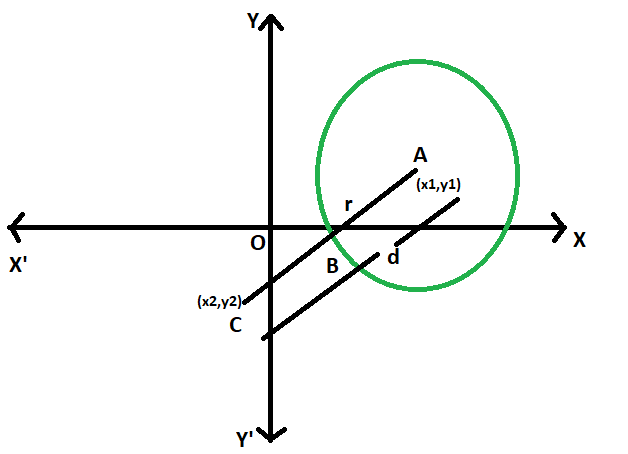Open in App
Not now

# Shortest distance between a point and a circle

• Last Updated : 07 Jun, 2022

Given a circle with a given radius has its centre at a particular position in the coordinate plane. In the coordinate plane, another point is given. The task is to find the shortest distance between the point and the circle.
Examples:

```Input: x1 = 4, y1 = 6, x2 = 35, y2 = 42, r = 5
Output: 42.5079

Input: x1 = 0, y1 = 0, x2 = 5, y2 = 12, r = 3
Output: 10```Approach:

• Let the radius of the circle = r

• co-ordinate of the centre of circle = (x1, y1)

• co-ordinate of the point = (x2, y2)

• let the distance between centre and the point = d

• As the line AC intersects the circle at B, so the shortest distance will be BC,
which is equal to (d-r)

• here using the distance formula,
d = √((x2-x1)^2 – (y2-y1)^2)

• so BC = √((x2-x1)^2 – (y2-y1)^2) – r

• so,Below is the implementation of the above approach:

## C++

 `// C++ program to find` `// the Shortest distance` `// between a point and` `// a circle` `#include ` `using` `namespace` `std;`   `// Function to find the shortest distance` `void` `dist(``double` `x1, ``double` `y1, ``double` `x2, ``double` `y2, ``double` `r)` `{` `    ``cout << ``"The shortest distance "` `         ``<< ``"between a point and a circle is "` `         ``<< ``sqrt``((``pow``((x2 - x1), 2))` `                 ``+ (``pow``((y2 - y1), 2)))` `                ``- r` `         ``<< endl;` `}`   `// Driver code` `int` `main()` `{` `    ``double` `x1 = 4, y1 = 6,` `           ``x2 = 35, y2 = 42, r = 5;` `    ``dist(x1, y1, x2, y2, r);` `    ``return` `0;` `}`

## Java

 `// Java program to find` `// the Shortest distance` `// between a point and` `// a circle` `class` `GFG` `{`   `// Function to find the shortest distance` `static` `void` `dist(``double` `x1, ``double` `y1, ``double` `x2,` `                                ``double` `y2, ``double` `r)` `{` `    ``System.out.println(``"The shortest distance "` `            ``+ ``"between a point and a circle is "` `            ``+ (Math.sqrt((Math.pow((x2 - x1), ``2``))` `                    ``+ (Math.pow((y2 - y1), ``2``)))` `            ``- r));` `}`   `// Driver code` `public` `static` `void` `main(String[] args)` `{` `    ``double` `x1 = ``4``, y1 = ``6``,` `            ``x2 = ``35``, y2 = ``42``, r = ``5``;` `    ``dist(x1, y1, x2, y2, r);` `}` `}`   `/* This code contributed by PrinciRaj1992 */`

## Python3

 `# Python program to find ` `# the Shortest distance ` `# between a point and ` `# a circle ` ` `  `# Function to find the shortest distance ` `def` `dist(x1, y1, x2, y2, r): ` `    ``print``(``"The shortest distance between a point and a circle is "` `    ``,((((x2 ``-` `x1)``*``*` `2``) ``+` `((y2 ``-` `y1)``*``*` `2``))``*``*``(``1``/``2``)) ``-` `r);`   ` `  `# Driver code ` `x1 ``=` `4``;` `y1 ``=` `6``; ` `x2 ``=` `35``;` `y2 ``=` `42``;` `r ``=` `5``; ` `dist(x1, y1, x2, y2, r); `     `# This code has been contributed by 29AjayKumar`

## C#

 `// C# program to find the Shortest distance` `// between a point and a circle` `using` `System;`   `class` `GFG` `{`   `// Function to find the shortest distance` `static` `void` `dist(``double` `x1, ``double` `y1, ``double` `x2,` `                                ``double` `y2, ``double` `r)` `{` `    ``Console.WriteLine(``"The shortest distance "` `            ``+ ``"between a point and a circle is "` `            ``+ (Math.Sqrt((Math.Pow((x2 - x1), 2))` `                    ``+ (Math.Pow((y2 - y1), 2)))` `            ``- r));` `}`   `// Driver code` `public` `static` `void` `Main(String[] args)` `{` `    ``double` `x1 = 4, y1 = 6,` `            ``x2 = 35, y2 = 42, r = 5;` `    ``dist(x1, y1, x2, y2, r);` `}` `}`   `/* This code contributed by PrinciRaj1992 */`

## PHP

 ``

## Javascript

 ``

Output:

`The shortest distance between a point and a circle is 42.5079`

Time Complexity: O(1)

Auxiliary Space: O(1)

My Personal Notes arrow_drop_up
Related Articles